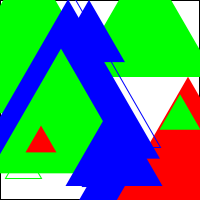PasteRack.org Paste # 71076 2019-01-22 17:42:22 Fork as a new paste. Paste viewed 412 times. Tweet Embed:
1. #lang racket
2.
3. ;Section 2
4.
5. (define pi 3.14159265)
6. (define (cylinder-surface-area r h)
7.   (+
8.    (* 2 pi r h)
9.    (* 2 pi r r)
10.   )
11.   )
12.
13. (define (max3 a b c)
14.   (cond
15.     [(and (>= a b) (>= a c)) a]
16.     [(and (>= b a) (>= b c)) b]
17.     [else c]
18.   )
19. )
20.
21. (define (rac list)
22.   (car (reverse list))
23.   )
24.
25. (define (rdc list)
26.   (reverse (cdr (reverse list)))
27.   )
28.
29. (define (snoc list1 list2)
30.  (reverse (cons list1 (reverse list2)) )
31.   )
32.
33.
34. (define (rnd-elmt list)
35.   (list-ref list (random (length list)))
36.   )
37.
38. ;Section 3
39.
40. (define (power-of-two? n)
41.   (cond
42.     [(< n 2) #f]
43.     [(= n 2) #t]
44.     [(even? n) (power-of-two? (/ n 2))]
45.     [else #f]
46.     )
47.   )
48. (define (pot? n) (power-of-two? n))
49.
50. ;Section 4
51. (require 2htdp/image)
52.
53. (define (circ d color) (circle (/ d 1) "solid" color))
54.
55.
56. (define (snowman d1 d2 d3 color)
57.   (overlay/offset (circ d1 color) 0 (+ d1 d2 d3) (overlay/offset (circ d2 color) 0 (+ d2 d3) (circ d3 color)))
58.   )
59.
60. (define (rnd-color) (rnd-elmt '("red" "blue" "green")))
61. (define (rnd-fill) (rnd-elmt '("solid" "outline")))
62.
63. (define (rnd-triangle maxsize) (triangle (random maxsize) (rnd-fill) (rnd-color)))
64.
65.
66. ;rnd-pic
67. (define (sqr-scene size) (empty-scene size size))
68.
69. (define (add-triangle scene size) (place-image (rnd-triangle size) (random size) (random size) scene))
70.
71. (define (rnd-pic n size)
72.         (cond
73.                 [(<= n 0) (sqr-scene size)]
74.                 [else (add-triangle (rnd-pic (sub1 n) size) size)]
75.         )
76. )
77.
78. ;Section 5
79.
80. (define (! n)
81.         (cond
82.                 [(<= n 0) 1]
83.                 [else (* n (! (sub1 n)))]
84.         )
85. )
86.
87. (define (exp m n)
88.         (cond
89.                 [(<= n 0) 1]
90.                 [else (* m (exp m (sub1 n)))]
91.         )
92. )
93.
94. (define (arctan x n)
95.         (cond
96.                 [(<= n 0) x]
97.                 [else (+ (arctan x (sub1 n))
98.                                 (* (exp -1 n)
99.                                         (/ (exp x (+ (* 2 i) 1))
100.                                            (+ (* 2 i) 1)
101.                                         )
102.                                 )
103.                           )
104.             ]
105.         )
106. )
107.
108. (define (myPI)
109.                 (exact->inexact (-
110.                                                         (* 16 (arctan 1/5 10))
111.                                                         (* 4 (arctan 1/239 10))
112.                                                 )
113.                 )
114. )
115.
116. ;Section 6
117.
118. (define (prod A)
119.         (cond
120.                 [(= (length A) 0) 1]
121.                 [else (* (car A) (prod (cdr A)))]
122.         )
123. )
124.
125. (define (>=0 l)
126.         (cond
127.                 [(= (length A) 0) #t]
128.                 [(< (car A) 0) #f]
129.                 [else (>= 0 (cdr l))]
130.         )
131. )
132.
133. (define (err-msg) (1))
134.
135. (define (nth n l)
136.         (cond
137.                 [(< n 0) (err-msg)]
138.                 [(> n (length l)) (err-msg)]
139.                 [(= n 0) (car l)]
140.                 [else (nth (sub1 n) (cdr l))]
141.         )
142. )
143.
144. (define (ordered l)
145.         (cond
146.                 [(<= (length l) 1) #t]
147.                 [(> (nth 1 l) (nth 0 l)) #f]
148.                 [else (ordered (cdr l))]
149.         )
150. )
151.
152. (define (max-err) (1))
153.
154. (define (maxlist l curMax)
155.         (cond
156.                 [(= 0 (length l)) curMax]
157.                 [(> (car l) curMax) (maxlist (cdr l) (car l))]
158.                 [else (maxlist (cdr l) curMax)]
159.         )
160. )
161.
162.
163.
164.
165. (define (max-list l)
166.         (cond
167.                 [(<= (length l) 0) (max-err)]
168.                 [else (maxlist l (car l))]
169.         )
170. )
171.
172. (rnd-pic 20 200)
173. (arctan 1/239 10)
174. (myPI)
175. (prod '(1))
176. (prod '(1 2))
177. (prod '(1 2 3 4))
178.
179. (>=0 '(0 4 6 1 5))
180. (>=0 '(0 4 6 1 -5))
181.
182. (nth 5 '(a b c d e f g))
183. (nth 0 '(a b c d e f g))
184. (nth 10 '(a b c d e f g))
185.
186. (ordered '())
187. (ordered '(1))
188. (ordered '(1 2))
189. (ordered '(2 1))

=>

exp: arity mismatch;

the expected number of arguments does not match the given

number

expected: 1

given: 2

arguments...:

-1

0

exp: arity mismatch;

the expected number of arguments does not match the given

number

expected: 1

given: 2

arguments...:

-1

0

1

2

24

A: undefined;

cannot reference an identifier before its definition

in module: 'm

A: undefined;

cannot reference an identifier before its definition

in module: 'm

'f

'a

application: not a procedure;

expected a procedure that can be applied to arguments

given: 1

arguments...: [none]

#t

#t

#f

#t

1.
2. ;Section 2
3.
4. (define pi 3.14159265)
5. (define (cylinder-surface-area r h)
6.   (+
7.    (* 2 pi r h)
8.    (* 2 pi r r)
9.   )
10.   )
11.
12. (define (max3 a b c)
13.   (cond
14.     [(and (>= a b) (>= a c)) a]
15.     [(and (>= b a) (>= b c)) b]
16.     [else c]
17.   )
18. )
19.
20. (define (rac list)
21.   (car (reverse list))
22.   )
23.
24. (define (rdc list)
25.   (reverse (cdr (reverse list)))
26.   )
27.
28. (define (snoc list1 list2)
29.  (reverse (cons list1 (reverse list2)) )
30.   )
31.
32.
33. (define (rnd-elmt list)
34.   )
35.
36. ;Section 3
37.
38. (define (power-of-two? n)
39.   (cond
40.     [(< n 2) #f]
41.     [(= n 2) #t]
42.     [(even? n) (power-of-two? (/ n 2))]
43.     [else #f]
44.     )
45.   )
46. (define (pot? n) (power-of-two? n))
47.
48. ;Section 4
49. (require 2htdp/image)
50.
51. (define (circ d color) (circle (/ d 1) "solid" color))
52.
53.
54. (define (snowman d1 d2 d3 color)
55.   (overlay/offset (circ d1 color) 0 (+ d1 d2 d3) (overlay/offset (circ d2 color) 0 (+ d2 d3) (circ d3 color)))
56.   )
57.
58. (define (rnd-color) (rnd-elmt '("red" "blue" "green")))
59. (define (rnd-fill) (rnd-elmt '("solid" "outline")))
60.
61. (define (rnd-triangle maxsize) (triangle (random maxsize) (rnd-fill) (rnd-color)))
62.
63.
64. ;rnd-pic
65. (define (sqr-scene size) (empty-scene size size))
66.
67. (define (add-triangle scene size) (place-image (rnd-triangle size) (random size) (random size) scene))
68.
69. (define (rnd-pic n size)
70.         (cond
71.                 [(<= n 0) (sqr-scene size)]
72.                 [else (add-triangle (rnd-pic (sub1 n) size) size)]
73.         )
74. )
75.
76. ;Section 5
77.
78. (define (! n)
79.         (cond
80.                 [(<= n 0) 1]
81.                 [else (* n (! (sub1 n)))]
82.         )
83. )
84.
85. (define (exp m n)
86.         (cond
87.                 [(<= n 0) 1]
88.                 [else (* m (exp m (sub1 n)))]
89.         )
90. )
91.
92. (define (arctan x n)
93.         (cond
94.                 [(<= n 0) x]
95.                 [else (+ (arctan x (sub1 n))
96.                                 (* (exp -1 n)
97.                                         (/ (exp x (+ (* 2 i) 1))
98.                                            (+ (* 2 i) 1)
99.                                         )
100.                                 )
101.                           )
102.             ]
103.         )
104. )
105.
106. (define (myPI)
107.                                                         (* 16 (arctan 1/5 10))
108.                                                         (* 4 (arctan 1/239 10))
109.                                                 )
110.                 )
111. )
112.
113. ;Section 6
114.
115. (define (prod A)
116.         (cond
117.                 [(= (length A) 0) 1]
118.                 [else (* (car A) (prod (cdr A)))]
119.         )
120. )
121.
122. (define (>=0 l)
123.         (cond
124.                 [(= (length A) 0) #t]
125.                 [(< (car A) 0) #f]
126.                 [else (>= 0 (cdr l))]
127.         )
128. )
129.
130. (define (err-msg) (1))
131.
132. (define (nth n l)
133.         (cond
134.                 [(< n 0) (err-msg)]
135.                 [(> n (length l)) (err-msg)]
136.                 [(= n 0) (car l)]
137.                 [else (nth (sub1 n) (cdr l))]
138.         )
139. )
140.
141. (define (ordered l)
142.         (cond
143.                 [(<= (length l) 1) #t]
144.                 [(> (nth 1 l) (nth 0 l)) #f]
145.                 [else (ordered (cdr l))]
146.         )
147. )
148.
149. (define (max-err) (1))
150.
151. (define (maxlist l curMax)
152.         (cond
153.                 [(= 0 (length l)) curMax]
154.                 [(> (car l) curMax) (maxlist (cdr l) (car l))]
155.                 [else (maxlist (cdr l) curMax)]
156.         )
157. )
158.
159.
160.
161.
162. (define (max-list l)
163.         (cond
164.                 [(<= (length l) 0) (max-err)]
165.                 [else (maxlist l (car l))]
166.         )
167. )
168.
169. (rnd-pic 20 200)
170. (arctan 1/239 10)
171. (myPI)
172. (prod '(1))
173. (prod '(1 2))
174. (prod '(1 2 3 4))
175.
176. (>=0 '(0 4 6 1 5))
177. (>=0 '(0 4 6 1 -5))
178.
179. (nth 5 '(a b c d e f g))
180. (nth 0 '(a b c d e f g))
181. (nth 10 '(a b c d e f g))
182.
183. (ordered '())
184. (ordered '(1))
185. (ordered '(1 2))
186. (ordered '(2 1))

=>exp: arity mismatch; the expected number of arguments does not match the given number expected: 1 given: 2 arguments...: -1 0 exp: arity mismatch; the expected number of arguments does not match the given number expected: 1 given: 2 arguments...: -1 0 1 2 24 A: undefined; cannot reference an identifier before its definition in module: 'm A: undefined; cannot reference an identifier before its definition in module: 'm 'f 'a application: not a procedure; expected a procedure that can be applied to arguments given: 1 arguments...: [none] #t #t #f #t